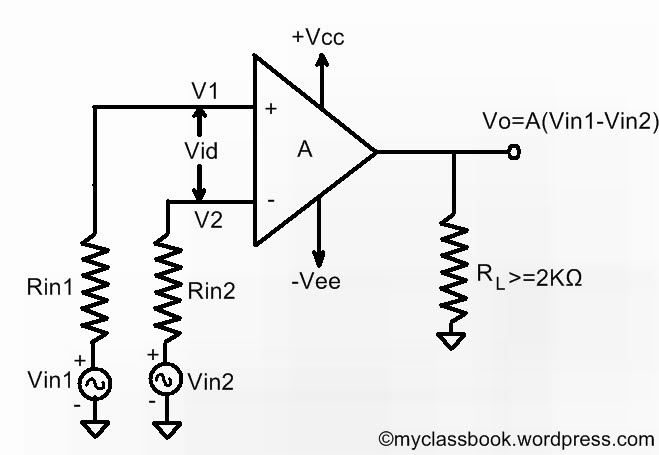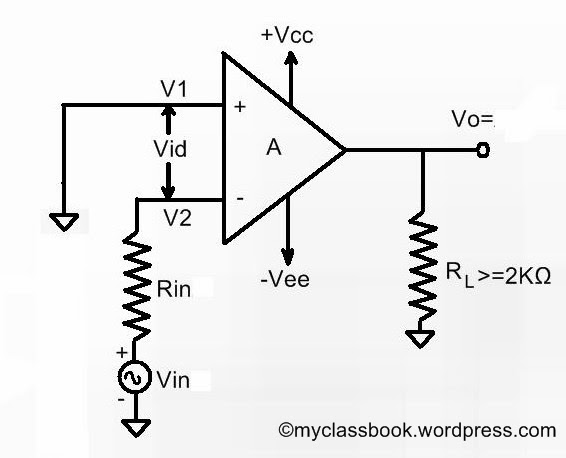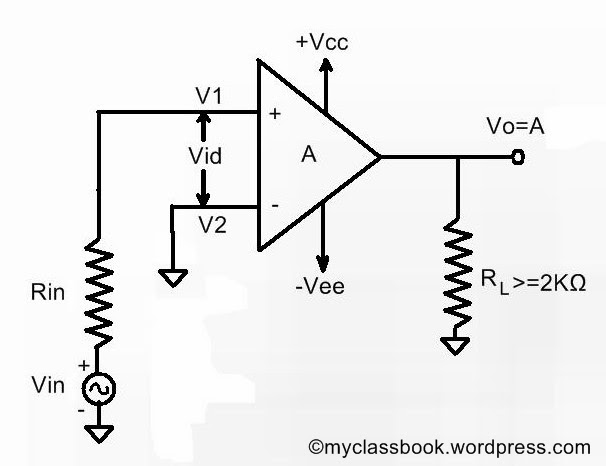# Differential, Inverting And Non-inverting Operational Amplifier

## Open loop OP-AMP Configurations:

In the case of amplifiers, the term open loop indicates that there is no connection, either direct or via another network, exists between the output and input terminals. That is the output signal is not fed back in any form as part of the input signal, and the loop that has would be formed with feedback is open.

When op-amp is connected in open loop configuration, it acts as high gain amplifier. There exist three open loop op-amp configurations as follows:

• 1)   Differential amplifier

• 2)   Inverting amplifier

• 3)   Noninverting amplifier

These three configurations are classed according to the number of inputs used and the terminal to which the input is applied when a single input is used.

## The differential amplifier:

The following figure shows the differential amplifier in which input signals Vin1 and Vin2 are applied to the positive and negative terminal of the op-amp respectively. As the op-amp amplifies the difference between the two input signals, this configuration is called ‘differential amplifier’.

[caption id="attachment_372" align="aligncenter" width="490"]Differential amplifier[/caption]

The op-amp is called versatile device because it amplifies both AC as well as DC input signals. The voltage drop across Rin1 and Rin2 can be neglected since they are very small as compared to input resistance of op-amp. Which then implies that V1=Vin1 and V2=Vin2.

Thus from the equation Vo=A(Vid)=A(V1-V2)

We get

Vo=A(Vin1-Vin2)

Thus the output voltage is equal to the voltage gain A times the difference between input voltages. The polarity of the output voltage depends upon the difference (Vin1-Vin2). In open loop configurations, the gain A is commonly referred as open-loop gain.

## The Inverting Amplifier:

[caption id="attachment_376" align="aligncenter" width="490"]Inverting Amplifier[/caption]

In inverting amplifier there is only one input which is applied to the inverting input terminal (which is negative). The Noninverting terminal is grounded.

Since V1=0V and V2=Vin

From the equation Vo=A(Vid)=A(Vin1-Vin2)

We get  Vo=-AVin

The negative sign shows that the output voltage is out of phase with respect to input by 180 degrees or is of opposite polarity.

## The Noninverting Amplifier:

The following figure shows the Noninverting amplifier. In this configuration, input is applied to the Noninverting input terminal, and the inverting terminal is connected to the ground.

[caption id="attachment_379" align="aligncenter" width="490"]Noninverting Amplifier[/caption]

Here we have V1=Vin  and V2=0V.

Therefore according to the equation Vo=A(Vid)=A(Vin1-Vin2)

We get Vo=AVin

It implies that the output voltage is voltage gain A times the applied voltage.